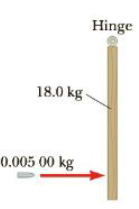Chapter 8, Problem 64P

Chapter
Section
Textbook Problem

A 0.005 00-kg bullet traveling horizontally with a speed of 1.00 × 103 m/s enters an 18.0-kg door, embedding itself 10.0 cm from the side opposite the lunges as in Figure P8.64. The 1.00-m-wide door is free to swing on its hinges, (a) Before it hits the door, does the bullet have angular momentum relative the door’s axis of rotation? Explain, (b) Is mechanical energy conserved in this collision? Answer without doing a calculation, (c) At what angular speed does the door swing open immediately after the collision? (The door has the same moment of inertia as a rod with axis at one end.) (d) Calculate the energy of the door-bullet system and determine whether it is less than or equal to the kinetic energy of the bullet before the collision.Figure P8.64 An overhead view of a bullet striking a door.

(a)

To determine

Whether the bullet has angular momentum before collision.

Explanation

The bullet travels with a horizontal speed.

At the instant just before the collision it can assume that the bullet is a body of mass mB traverse in a circular path of radius l which is the distance from the hinge to bullet, with a tangential speed v

The below figure shows the bullet just before the collision with the door

(b)

To determine

Whether mechanical energy is conserved in the collision.

(c)

To determine

The angular speed of the door.

(d)

To determine

Whether the kinetic energy of the bullet-door system is less than or equal to kinetic energy of the bullet before collision.

Still sussing out bartleby?

Check out a sample textbook solution.

See a sample solution

The Solution to Your Study Problems

Bartleby provides explanations to thousands of textbook problems written by our experts, many with advanced degrees!

Get Started

Find more solutions based on key concepts